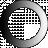# American Dictionary of the English Language

Dictionary Search

### Ratio

RA'TIO, noun ra'sho. [Latin from ratus, reor, to think or suppose, to set, confirm or establish. Reor is contracted from redor or retor, and primarily signifies to throw, to thrust, hence to speak, to set in the mind, to think, like Latin suppono; and setting gives the sense of a fixed rate or rule. See Reason.]

Proportion, or the relation of homogeneous things which determines the quantity of one from the quantity of another, without the intervention of a third.

The relation which one quantity has to another of the same kind, as expressed by the quotient of the one divided by the other. Thus the ratio of 4 to 2 is 4/2, or 2; and the ratio of 5 to 6 is 5/6. This is geometrical ratio which is that signified when the term is used without distinction; but arithmetical ratio is the difference between two quantities. Thus the arithmetical ratio of 2 to 6 is 4.

Ratio respects magnitudes of the same kind only. One line may be compared with another line, but a line cannot be compared with a superficies, and hence between a line and a superficies there can be no ratio